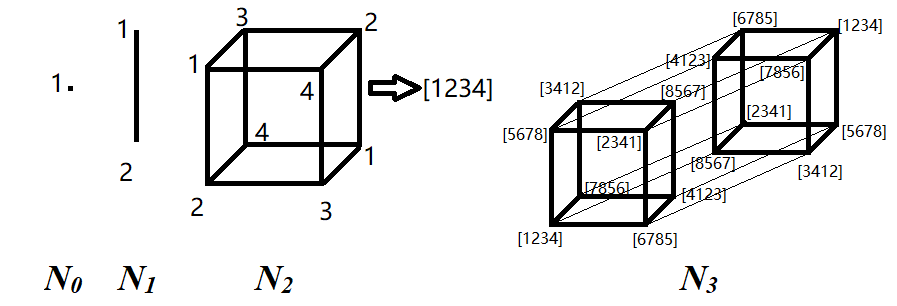# 题解与讨论liu_cheng_ao 2018-04-25 8:50:33 2018-07-29 8:59:07

### 问题

**这个问题是图论中控制数问题的延伸，关于这类问题已有很多研究。**竞赛中也有专门引入和研究过相关内容（如 IMO 2017 集训队讲课）。下面我们给出一个简单的 -近似构造方法。

### n=2^k-1 的构造（请结合这张图来理解）

#include<bits/stdc++.h>

using namespace std;

struct Cube{
int *a,n,c,N,NN;
Cube& operator <<= (const int x){
for(int i=0;i<NN;i++)a[i]=(a[i]+x)%c;
return *this;
}
Cube& operator += (const int x){
for(int i=0;i<NN;i++)a[i]+=x;
return *this;
}
Cube operator << (const int x){
Cube r=*this;
r <<= x;
return r;
}
Cube operator + (const int x){
Cube r=*this;
r += x;
return r;
}
int& operator [] (const int x){
return a[x];
}
Cube(){}
Cube(int _n){
n=_n;
c=1<<n;
N=(1<<n)-1;
NN=1<<N;
if(n==0){
a = new int[NN];
a = 0;
}else{
Cube x(n-1);
a = new int[NN];
for(int i=0,j=0,s=NN/2;i<x.NN;i++,j+=x.NN)
memcpy(a+j,((x<<x[i])+(c/2)*(__builtin_popcount(j)&1)).a,sizeof(int)*x.NN),
memcpy(a+s+j,((x<<x[i])+(c/2)*(__builtin_popcount(s+j)&1)).a,sizeof(int)*x.NN);
x.print();
}
}
Cube(const Cube& x){
n=x.n,c=x.c,N=x.N,NN=x.NN;
a = new int[NN];
memcpy(a,x.a,sizeof(int)*NN);
}
~Cube(){
delete[] a;
}
void print(){
for(int i=0;i<NN;i++)cout<<a[i]<<' ';
cout<<endl;
}
};

int main(){
Cube(3).print(); // 本题 1 到 7 号测试点只需输出一个含 N3 子空间的超立方体即可
return 0;
}


### 共 2 条回复